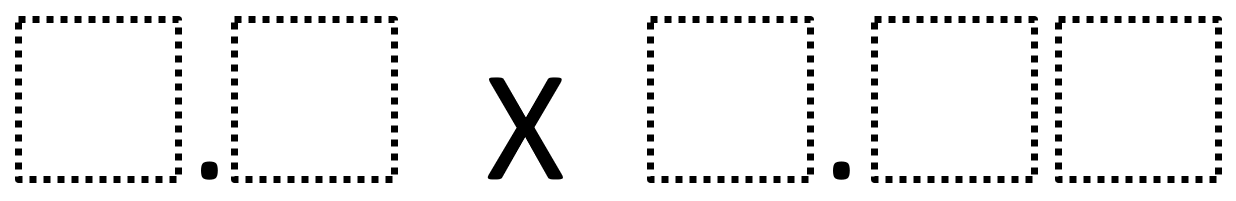# Multiplying Decimals to Make a Whole Number Product

Directions: Using the digits 1 to 9 at most one time each, fill in the boxes to make a whole number product.### Hint

How can we tell if it is even possible to make a whole number product?
What digits would be better or worse choices for making a whole number product?

Number of Unique Solutions: 14
1: 1.6 x 3.75 = 6
2: 1.6 x 8.75 = 14
3: 2.4 x 3.75 = 9
4: 2.4 x 8.75 = 21
5: 3.2 x 8.75 = 28
6: 4.8 x 1.25 = 6
7: 4.8 x 3.75 = 18
8: 4.8 x 6.25 = 30
9: 6.4 x 1.25 = 8
10: 6.4 x 3.75 = 24
11: 6.4 x 8.75 = 56
12: 9.6 x 1.25 = 12
13: 9.6 x 3.75 = 36
14: 9.6 x 8.75 = 84
Note what they all have in common!

Source: Owen Kaplinsky

## Equivalent Expressions with Fractions

Directions: Using the digits 0 to 9 at most one time each and choosing either …

1.another one… 9.6 x 1.25 = 12

2.3.Hi
If you think about multiplying integers, you need the product to have a factor of 1000. I.e. you need prime factors of the multipliers to include 5^3 and 2^3. Additionally, the factors of 5 and 2 must stay in separate multipliers otherwise one would have to end in zero which is not allowed.
So possible numbers that would work are:
1.6,2.4,3.2,4.8,5.6,6.4,7.2,9.6
multiplied by
1.25,3.75,6.25,8.75
Any combination of these will work, if the digits are unique.

4.3.75 X 4.8 =18 Also Works

5.•Ok I will.

6.6.25 x 4.8 = 30

7.I got an answer of 1.25 x 4.8 = 6

8.6.4 times 1.25 is another answer to get 8… from one of my amazing 5th grade students!

9.10.I am amazed that my high math students couldn’t figure it out. They got pretty frustrated. However, some of my lower students worked hard to figure it out.

•Problems like this make you re-evaluate the labels we give students including whether they’re true or useful.

11.I think this would be a wonderful investigation to use in the context of the book ‘The 5 Practices in Practice’ book. However, I am struggling to come up with a statement about ‘What am I wanting the students to learn?’

Any thoughts.

•Hi
For my sixth graders, my learning goal would be to practice the multiplication of a decimal by a decimal. It has them practicing more engaging way, and because they are problem solving and looking for patterns, they aren’t really aware of the boring repeated practice that a worksheet would have.

12.you might see a lot of answer in life.

13.My 5th grade students were excited to find the answer 9.6 * 8.75 = 84.